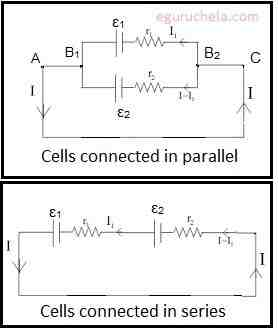# Cells in Series and in Parallel

When cells are connected in series with each other and they are all connected in the same direction the total potential difference supplied to the circuit is the individual potential differences added together.

Vtotal = V1 + V2 + V3 + ......
If the cells in parallel connection, the voltage cannot be increased by increasing the number of cells present in the circuit. It is because they do not have same circular path. In the case of parallel connection, the connection provides power based on one cell therefore brightness of the bulb will not get high.
The advantages of cells connected in parallel:
If any one of the cell gets damage in the circuit, it does not affect the whole connection. The cells connected in parallel last longer and do not exhaust easily.
The advantages of cells connected in series:
Cells connected in series, such circuits do not overheat easily and the design of series circuit is simple compared to parallel circuits. The greater resultant voltage get produced and damaged cells can be easily identified/replaced as they break the circuit.Case 1:
The cells connected in parallel V = ε1 r2 + ε2 r1/ r1+ r2 – Ir1r2 / r1+ r2.
V = εeq / req(where n number of cells connected in parallel)
= ε1 / r1 + ε2 / r2 +…………………….. εn / rn.
Case 2:
The cells connected in series the voltage developed is V = (ε1 + ε2) – I (r1+r2).
V = εeq - req (if replace the number of cells by a single cell)